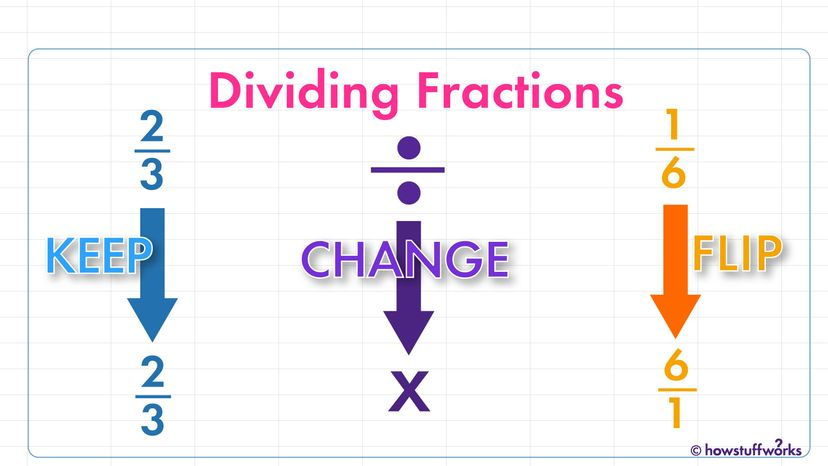# How to Divide Fractions

By: Jesslyn Shields  |Dividing fractions is easy if you just remember to keep, change and flip. HowStuffWorks

The words "dividing fractions" can cause anxiety in almost anyone — you have to flip fractions and know words like divisor and dividend and reciprocal. The steps involved in dividing fractions may seem hard to remember, but they're easy with a little practice. Because math is all about remembering rules and terms, and if you can do that, dividing fractions is a breeze.

Division is the inverse of multiplication, so one thing you have to remember when dividing fractions is the answer is always going to be larger than either of the components of the problem. You're basically trying to figure out how many of the divisor (the second number in the problem) can be found in the dividend (the first number). If you know how to multiply fractions, you'll have no trouble learning how to divide fractions.

Contents

## How Do You Divide Two Fractions?

### Step 1: Keep the Numerators and Denominators

Before you start, look at both your fractions, take a deep breath and tell yourself that if a sixth grader can learn how to divide fractions, you can become proficient at dividing fractions, too.

The first step to dividing fractions is just as simple as that little pep talk. Let's say you're trying to figure out the answer to 2/3 ÷ 1/6. Don't do anything! Keep the numerator and denominator of both numbers just as they are.

### Step 2: Change the Division Sign

The second step in dividing fractions is to multiply the two fractions. So, you simply have to change the division sign (÷) sign to a multiplication sign (x): 2/3 ÷ 1/6 becomes 2/3 x 1/6.

### Step 3: Flip the Second Fraction

The third step in dividing fractions is to find the reciprocal of the divisor — but don't panic! Dividing two fractions is the same as multiplying the first fraction by the reciprocal of the second fraction.

That just means you've got to flip the numerator (the top number) and the denominator (the bottom number) of the fraction on the right side of the division sign, which is called the divisor.

For instance, if you're dividing 2/3 by 1/6, you'd start by flipping the divisor: 2/3 x 6/1 = 12/3.

### Now You Have an Improper Fraction

You might notice that the fraction is no longer in proper fraction form, in which the numerator is smaller than the denominator; it's an improper fraction.

Improper fractions are those in which the number the fraction represents is larger than 1.

### Step 4: Is That Your Final Answer? No. Simplify the Fraction

All you need to do next is simplify the fraction 12/3. You do this by finding the largest number that divides equally into both the numerator and the denominator, which, in this case, is 3, which means the fraction simplifies to 4/1, or just 4. That's your final answer.Fractions simply represent all the parts that make up a whole. Chekyravaa/Shutterstock

## How Do You Divide Mixed Fractions?

Dividing fractions with mixed numbers is slightly different. You first have to convert the mixed fractions (fractions with whole numbers) to improper fractions and then divide them in the same way that you divided two fractions. Here is an example: 3/4 ÷ 1 1/2.

### Step 1: Convert Your Mixed Fraction to an Improper Fraction

So, the first step is to convert 1 1/2 to an improper fraction. 1 1/2 is the same as 3/2. Now, the problem can be solved like this: 3/4 ÷ 3/2.

### Step 2: Change the Division Sign

So, you simply have to change the division sign (÷) to a multiplication sign (x): 3/4 ÷ 3/2 becomes 3/4 x 3/2.

### Step 3: Flip the Second Fraction

Leave your first fraction as it is, but flip the second fraction, so 3/4 x 3/2 becomes 3/4 x 2/3 = 6/12.

### Step 4: Simplify the Fraction

From there, you just need to simplify: 6/12 = 1/2.

Therefore, the answer to the problem 3/4 ÷ 1 1/2 = 1/2.

So, to divide a mixed number with a fraction, first convert the mixed number to an improper fraction and follow the steps shown above.

## How Do You Divide Fractions With Different Denominators?

Now that we have all the basic terminology down and two examples under our belts, dividing fractions with different denominators is easy.

### Step 1: Find the Reciprocal of the Second Fraction

Simply reverse the numerator and denominator.